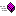XLL+ Class Library (7.0)

# Common Language Runtime support functions

Common Language Runtime support functions are listed here.

#### Excel to CLRtoClr Transform a value received from Excel to a CLR formtoClr Transform an array received from Excel to a CLR arraytoClrDate Transform an Excel date value to a CLR DateTime valuetoClrDates Transform an array of Excel date values to a CLR DateTime arraytoClrInts Transform an array of long to a CLR int arraytoClrObject Transform an Excel value of unknown type to a CLR objecttoClrObjectMatrix Transform an Excel matrix containing values of unknown type to a two-dimensional CLR object arraytoClrObjectVector Transform an Excel vector containing values of unknown type to a one-dimensional CLR object array

#### CLR to ExcelobjectToXl Transform a value of indeterminate type, received from .NET, to an Excel return valuetoXl Transform a value received from .NET to an Excel return valuetoXl Transform a value received from .NET to an Excel return value